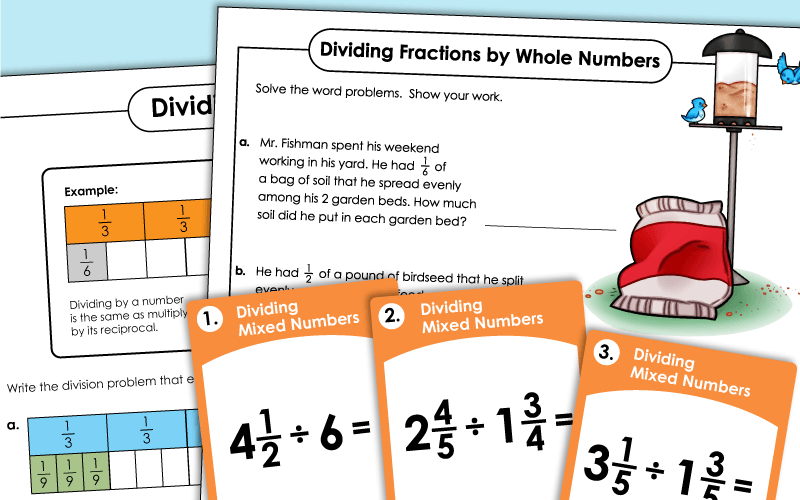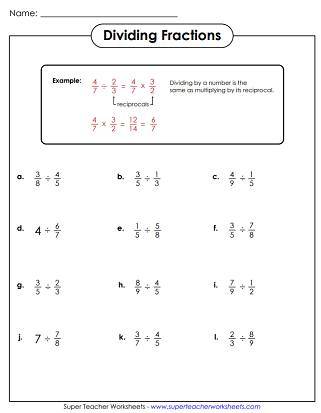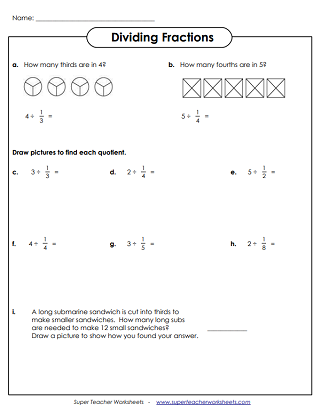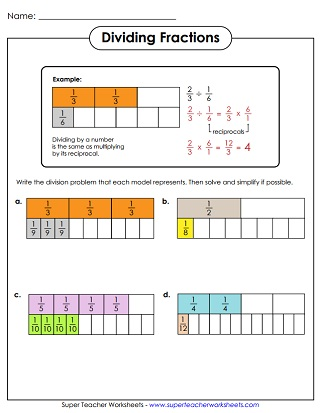# Dividing Fractions & Mixed Numbers

The printable worksheets below can be used to help students learn to divide fractions. Some worksheets include illustrations, diagrams and models. Others feature algorithm practice.## Dividing Fractions

Divide whole numbers by fractions.  Includes two illustrated problems and two word problems.
These printable task cards have division problems with fractions. Use these cards for small group instruction, classroom games, learning centers, or peer study sessions.

## Dividing Mixed Numbers

First, convert the mixed numbers into improper fractions. Then write the reciprocal of the divisor. Then multiply the numerators and denominators to find the quotient.
Here are 30 task cards with problems that require students to divide mixed numbers. Use these cards for extra practice, learning centers, or small group instruction. They also work great for whole-group instruction with a document camera.
Multiplying Fractions

These worksheets will help you teach your students to multiply fractions and mixed numbers. Many worksheets include graphical model problems and word problems.

Reciprocal Fractions

If you're teaching fraction division, you may also want to check out these worksheets on reciprocal fractions. Students find the reciprocals of fractions and mixed numbers.

More Fraction Worksheets

This page contains fraction addition, subtraction, multiplication, and division. Also includes operations with mixed numbers, equivalent fractions, simplifying fractions, and more.

## Sample Worksheet ImageMy Account
Site Information